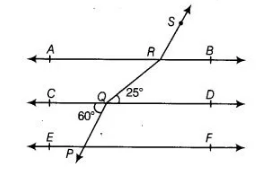# In figure, if AB || CD || EE, PQ || RS,`
Question:

In figure, if AB || CD || EE, PQ || RS, ∠RQD = 25° and ∠CQP = 60°, then ∠QRS is equal to(a) $85^{\circ}$

(b) $135^{\circ}$

(c) $145^{\circ}$

(d) $110^{\circ}$

Solution:

(c) Given, PQ || RS

∠PQC = ∠BRS = 60° [alternate exterior angles and ∠PQC = 60° (given)] and ∠DQR = ∠QRA = 25° [alternate interior angles]

[∠DQR = 25°, given]

∠QRS = ∠QRA + ∠ARS

= ∠QRA + (180° – ∠BRS) [linear pair axiom]

= 25° + 180° – 60°= 205° – 60°= 145°